# Steiner Triple System A27 Graph

The Second DIMACS Implementation Challenge: 1992-1993

Originator: Carlo Mannino

NP Hard Problems: Maximum Clique, Graph Coloring, and Satisfiability, The Second DIMACS Implementation Challenge: 1992-1993.

Clique formulation of the Steiner Triple Problem, translated from the set covering formulation.

## Examples

### Basic Examples

Retrieve the graph:

 In:=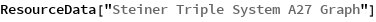Out=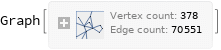Summary properties:

 In:=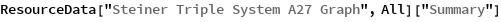Out=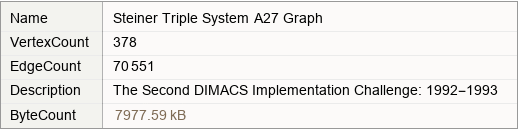### Basic Applications

Show the properties of the graph:

 In:=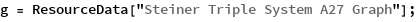In:=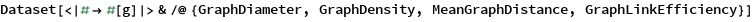Out=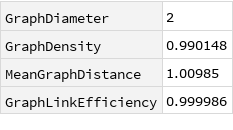Wolfram Research, "Steiner Triple System A27 Graph" from the Wolfram Data Repository (2019)